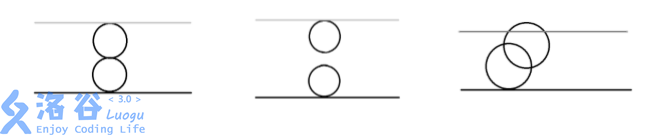P3958 奶酪

• 11K通过
• 46.9K提交
• 题目提供者 CCF_NOI
• 评测方式 云端评测
• 标签 并查集 广度优先搜索,BFS 搜索 NOIp提高组 2017
• 难度 普及/提高-
• 时空限制 1000ms / 256MB
• 提示：收藏到任务计划后，可在首页查看。

题目描述

现有一块大奶酪，它的高度为 $h$，它的长度和宽度我们可以认为是无限大的，奶酪 中间有许多 半径相同 的球形空洞。我们可以在这块奶酪中建立空间坐标系，在坐标系中， 奶酪的下表面为$z = 0$，奶酪的上表面为$z = h$。

现在，奶酪的下表面有一只小老鼠 Jerry，它知道奶酪中所有空洞的球心所在的坐 标。如果两个空洞相切或是相交，则 Jerry 可以从其中一个空洞跑到另一个空洞，特别 地，如果一个空洞与下表面相切或是相交，Jerry 则可以从奶酪下表面跑进空洞；如果 一个空洞与上表面相切或是相交，Jerry 则可以从空洞跑到奶酪上表面。

位于奶酪下表面的 Jerry 想知道，在 不破坏奶酪 的情况下，能否利用已有的空洞跑 到奶酪的上表面去?

空间内两点$P_1(x_1,y_1,z_1)$、$P2(x_2,y_2,z_2)$的距离公式如下：

$$\mathrm{dist}(P_1,P_2)=\sqrt{(x_1-x_2)^2+(y_1-y_2)^2+(z_1-z_2)^2}$$

输入输出格式

输入格式：

每个输入文件包含多组数据。

的第一行，包含一个正整数 $T$，代表该输入文件中所含的数据组数。

接下来是 $T$ 组数据，每组数据的格式如下： 第一行包含三个正整数 $n,h$ 和 $r$，两个数之间以一个空格分开，分别代表奶酪中空 洞的数量，奶酪的高度和空洞的半径。

接下来的 $n$ 行，每行包含三个整数 $x,y,z$，两个数之间以一个空格分开，表示空 洞球心坐标为$(x,y,z)$。

输出格式：

$T$ 行，分别对应 $T$ 组数据的答案，如果在第 $i$ 组数据中，Jerry 能从下 表面跑到上表面，则输出Yes，如果不能，则输出No （均不包含引号）。

输入输出样例

输入样例#1： 复制
3
2 4 1
0 0 1
0 0 3
2 5 1
0 0 1
0 0 4
2 5 2
0 0 2
2 0 4
输出样例#1： 复制
Yes
No
Yes

说明

【输入输出样例 1 说明】第一组数据,由奶酪的剖面图可见：

第一个空洞在$(0,0,0)$与下表面相切

第二个空洞在$(0,0,4)$与上表面相切 两个空洞在$(0,0,2)$相切

输出 Yes

第二组数据,由奶酪的剖面图可见：

两个空洞既不相交也不相切

输出 No

第三组数据,由奶酪的剖面图可见：

两个空洞相交 且与上下表面相切或相交

输出 Yes

【数据规模与约定】

对于 $20\%$的数据,$n = 1$,$1 \le h$ , $r \le 10,000$,坐标的绝对值不超过 $10,000$。

对于 $40\%$的数据,$1 \le n \le 8$, $1 \le h$ , $r \le 10,000$,坐标的绝对值不超过 $10,000$。

对于$80\%$的数据, $1 \le n \le 1,000$, $1 \le h , r \le 10,000$,坐标的绝对值不超过$10,000$。

对于 $100\%$的数据,$1 \le n \le 1,000$,$1 \le h , r \le 1,000,000,000$,$T \le 20$,坐标的 绝对值不超过 $1,000,000,000$。

提示
标程仅供做题后或实在无思路时参考。
请自觉、自律地使用该功能并请对自己的学习负责。
如果发现恶意抄袭标程，将按照I类违反进行处理。